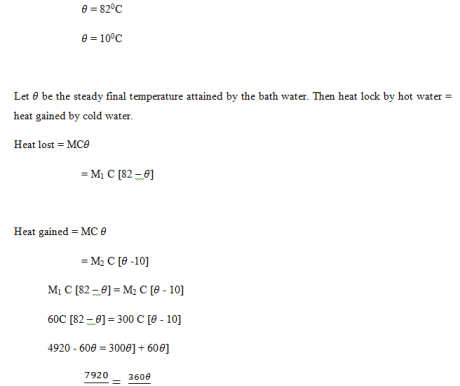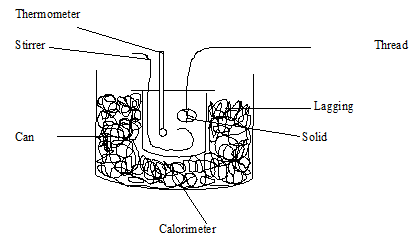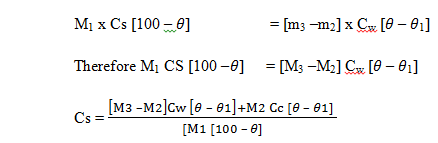# PHY4: HEAT CAPACITIES

##### This Unit is about Heat capacity

HEAT CAPACITIES

Heat capacity [c] of a body is defined as the quantity of heat required to raise its temperature by 1 k. The S.1 unit of heat capacity is the joule per kelvin [JK-1]

Note

Heat transfer causes a temperature change

The temperature change caused depends on;-

1. The mass of the substance being heated i.e. the bigger the mass the smaller the temperature change
2. The material of substance for example aluminum material of the same mass needs twice the amount of heat as iron material to rise through the same temperature.

Note

The quantity of heat acquired or given out by a body is the product of the heat capacity of the body and the temperature change.Specific heat capacity [c]

Is defined as the quality of heat required to raise the temperature of one kilogram of a body or a substance by 1k. The s.1 unit is joule per – kilogram per Kelvin [Jkg -1K-1]

The quality of heat gained or lost by a body is the product of its mass by the specific heat capacity and the temperature change

Q = mass X s.h.c X temperature change.The specific heat capacities of water and ice are 4200 Jkg-1K and 2100 Jkg -1K-1.

Example

1. An immersion heater is used to heat 500 grams of water in a polystyrene pot. If the temperature raises after 600 seconds in 140 c, calculate the power ratio of the heater given that the S.H.C of water is 4200 Jkg -1K-1

C = 4200 Jkg -1 K-1

M = 500 g

= 0.5 kg60kg of hot water at 82oc is run into a bath and is found to be too hot. If 300kg of cold water at 100c is added to lower the temperature. Calculate the final temperature of the both water.

M1 = 60kg

M2 = 300kgExperiment to determine the specific heat capacities of solids by method of mixturesNote

Heat flows from a hot object to a cold object until the temperatures of the objects are equal. This is true if there no heat losses to the surrounding thus heat lost by the hot object is equal to heat gained by the cold object.

During the method of mixture, the cold object is usually water and the hot object is the substance whose specific heat capacity is being determined.

The mass of such a solid is found [m1]. A string is then tied around the solid and placed in boiling water. The mass of the calorimeter [m2] is also determined and some cold water is added to the calorimeter which is just enough to cover the solid. The mass of the calorimeter and the water is determined [m3].

Record the temperature of the water and let it be 1. Put thick lagging around the calorimeter [cotton wool] lift the hot solid out of the boiling water and shake it to remove any water from it.

Quickly and gently lower the solid into the cold water in the calorimeter, stir the water well and record the highest temperature  reached by the mixture.

Heat loss by solid = heat gained by water + calorimeterA graph of temperature against time [shows variation]VIDEO EXPLAINS MORE ABOUT HEAT CAPACITY

## STEM Elearning

We at FAWE have built this platform to aid learners, trainers and mentors get practical help with content, an interactive platform and tools to power their teaching and learning of STEM subjects, more